# Electrical Engineering test questions for exams and entrance - 4

## Electrical Engineering test questions for exams and entrance - 4

(1) For a transmission line, if the magnitude of open circuit input impedance is 100 Ω and magnitude of short circuit input impedance is 25 Ω then the characteristic impedance

of transmission line will be

(A) 10 Ω
(B) 20 Ω
(C) 100 Ω
(D) 50 Ω

(2) What are the Eigen values of matrix A given below?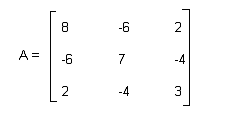(A) 0, 8, 15
(B) 0, 7, 11
(C) 0, 3, 15
(D) 0, 5,18

(3) Figure shows the ring oscillator. 100pico sec is the propagation delay of each inverter. The fundamental frequency of the oscillator output is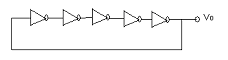(A) 1 GHz
(B) 100 MHz
(C) 1 MHz
(D) 20 GHz

(4) Consider a 3-phase transformer with the rating of 20MVA, 220KV (star) – 33KV (delta) with leakage reactance of 12%.What should be the transformer reactance when referred to each phase

of the L.V. delta connected side?

(A) 15.4
(B) 18.6
(C) 1.5
(D) 19.6

(5) Let D be the constant duty ratio of a step down chopper, which is operated in the continuous conduction mode in steady state. Vo = Magnitude of the dc output voltage and Vs =

Magnitude of the dc input voltage. The ratio Vo/Vs is given by

(A) 1/D
(B) D
(C) 1-D
(D) 1/1-D

(6) How is the feed water coming to the economizer in a thermal power plant is heated?

(A) Flue gases
(B) H.P stream
(C) L.P stream
(D) Directly heating the furnace

(7) In high voltage overhead transmission lines, bundled conductors are mainly used to

(A) Increase mechanical strength of the line
(B) Reduce loss in the transmission lines
(C) Reduce sag
(D) Reduce corona

(8) s-1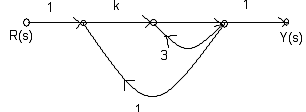What should be the value of k for the system to remain stable?

(A) 1< k < 3
(B) k < -1
(C) k > 3
(D) 1 < k < -1

(9) Consider a p-pole machine where θe is the electrical degree and ?m is the mechanical degree. The relation between θe and θm is given by

(A) θe = p/2 θm
(B) θe = 2/p θm
(C) θe = θm
(D) θe = p θm

(10) In order to protect the transformers, harmonic restraint is used to

(A) To guard against lightning
(B) To guard against magnetizing inrush current
(C) To guard against switching over voltages
(D) To guard against unbalanced operation

ANSWER: To guard against magnetizing inrush current

(12) Consider a dc generator operating on load. The brushes of generator are on the geometrical neutral axis (GNA). The magnetic neutral axis (MNA) is shifted in the direction of rotation.

What will happen if the brushes are given a lead of 90° (electrical)?

(A) The MNA will shift forward by less than 90°
(B) The MNA will shift forward by more than 90°
(C) The MNA will coincide with GNA
(D) The MNA will shift forward by 90°

ANSWER: The MNA will coincide with GNA

(13) What will be the common mode rejection ratio (CMRR) of an op-amp if the common mode gain is 0.01 and differential mode gain is 105?

(A) 10-7
(B) 107
(C) 109
(D) 10-9

(14) Let an electric train is driven by a dc series motor that faces a constant power load. It is running at rated speed and rated voltage. In order to bring down the speed to 0.25 p.u.

the supply voltage has to be brought down to

(A) 0.5p.u.
(B) 0.25p.u.
(C) 0.75p.u.
(D) 1.5p.u.

(15) In the output of _________ type of power amplifier crossover distortion is exhibited.

(A) Class C
(B) Class AB
(C) Class A
(D) Class B

(16) Consider a relay with a negative sequence whose plug setting is 0.2 A. For the operation of relay the minimum value of line to line fault current when the current transformer ratio is

5:1, is

(A) 0.245A
(B) 0.543A
(C) 1.732A
(D) 1/1.732A

For Questions 17 and 18 refer to the figure given below:

A unity feedback closed loop control system is shown in the figure.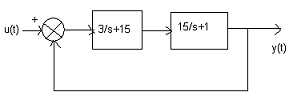(17) To the unit step input, the steady state error in the response is

(A) 50%
(B) 25%
(C) 30%
(D) 1%

(18) The roots of the closed loop characteristic equation of the system are

(A) 2 and -7
(B) -3 and 8
(C) -6 and 10
(D) -1 and 15

(19) Total 8 memory chips are present in a memory system. Each memory chips has 12 address lines and 4 data lines. The total size of the memory system is

(A) 32 K bytes
(B) 16 K bytes
(C) 48 K bytes
(D) 64 K bytes

(20) What will be the corona loss on a particular system at 60 Hz if the corona loss at 50 Hz is 1Kw/km per phase?

(A) 1.23 Kw/km per phase
(B) 1.13 Kw/km per phase
(C) 2 Kw/km per phase
(D) 1.25 Kw/km per phase

(21) When all the input of a logic gate is “0”, the output is “1”. The type of gate is either

(A) A NOR or an EX-NOR
(B) A NAND or an EX-OR
(C) An AND or a NOR
(D) A NAND or a NOR

ANSWER: A NOR or an EX-NOR

(22) For a bulb, the hot resistance of the filament is higher than the cold resistance. Give reason.

(A) Temperature coefficient of the filament is infinite.
(B) Temperature coefficient of the filament is zero
(C) Temperature coefficient of the filament is negative
(D) Temperature coefficient of the filament is positive

ANSWER: Temperature coefficient of the filament is positive

(23) Figure shows the 4-to-1 MUX. The output f is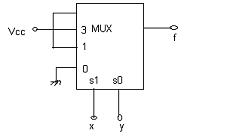(A) xy + x
(B) xy + y
(C) x + y
(D) None of the above

(24) Consider a dc series motor that is fed from a rated supply voltage. It is overloaded and its magnetic circuit is saturated. Which curve represents the torque speed characteristic of

this motor?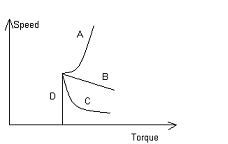(A) Curve D
(B) Curve C
(C) Curve B
(D) Curve A

(25) What will happen if diameter of a λ/2 dipole antenna is increased from λ/100 to λ/50?

(A) Gain decreases
(B) Gain increases
(C) Bandwidth decreases
(D) Bandwidth increases